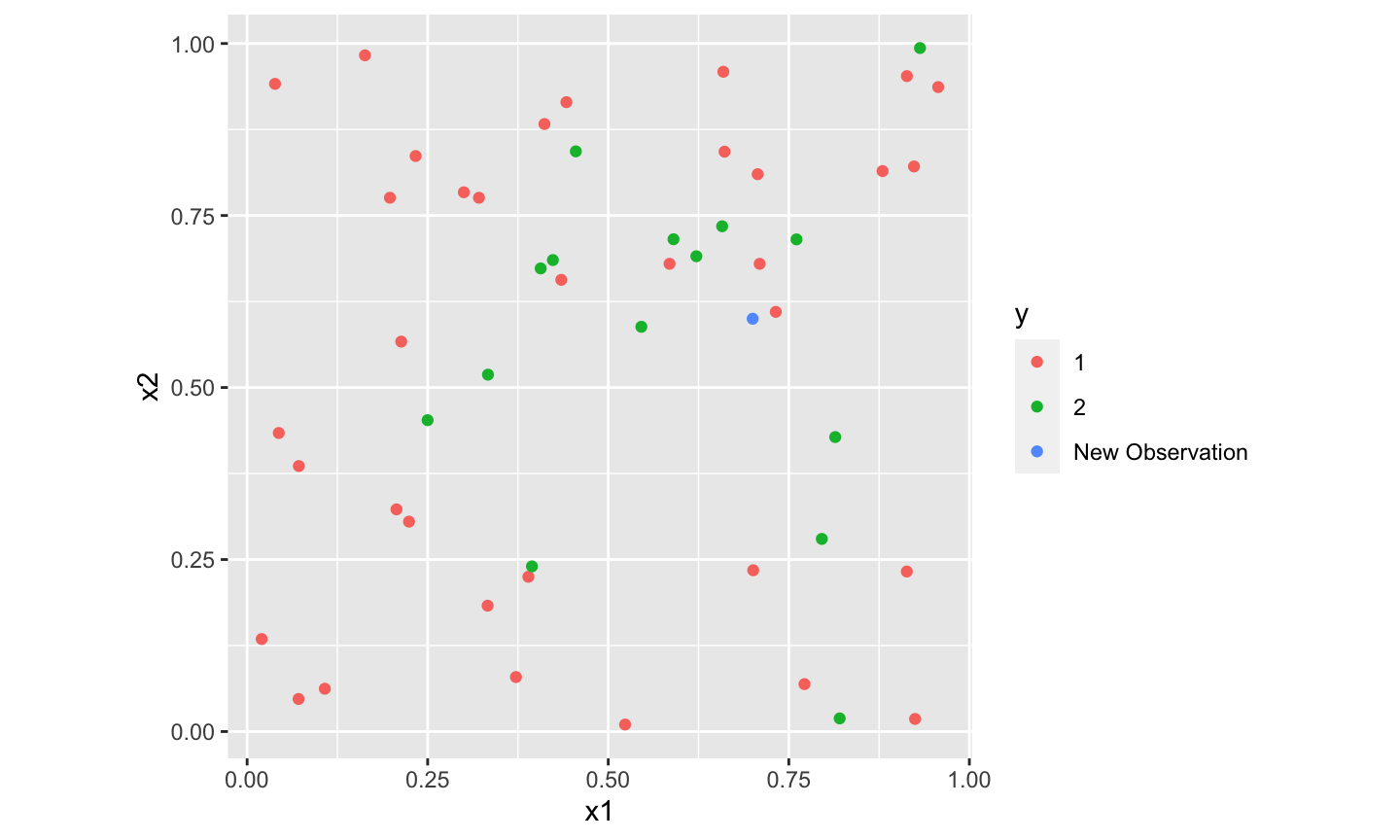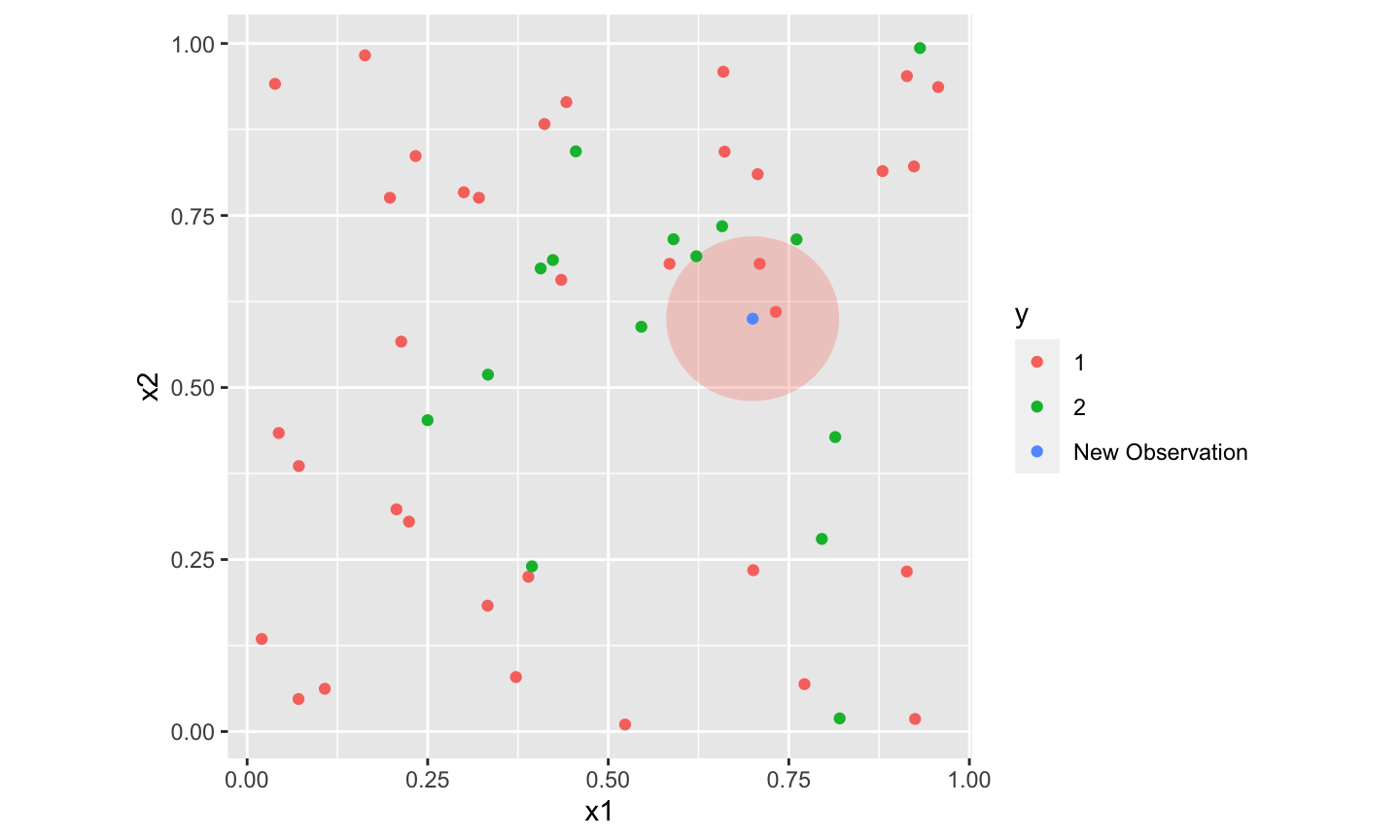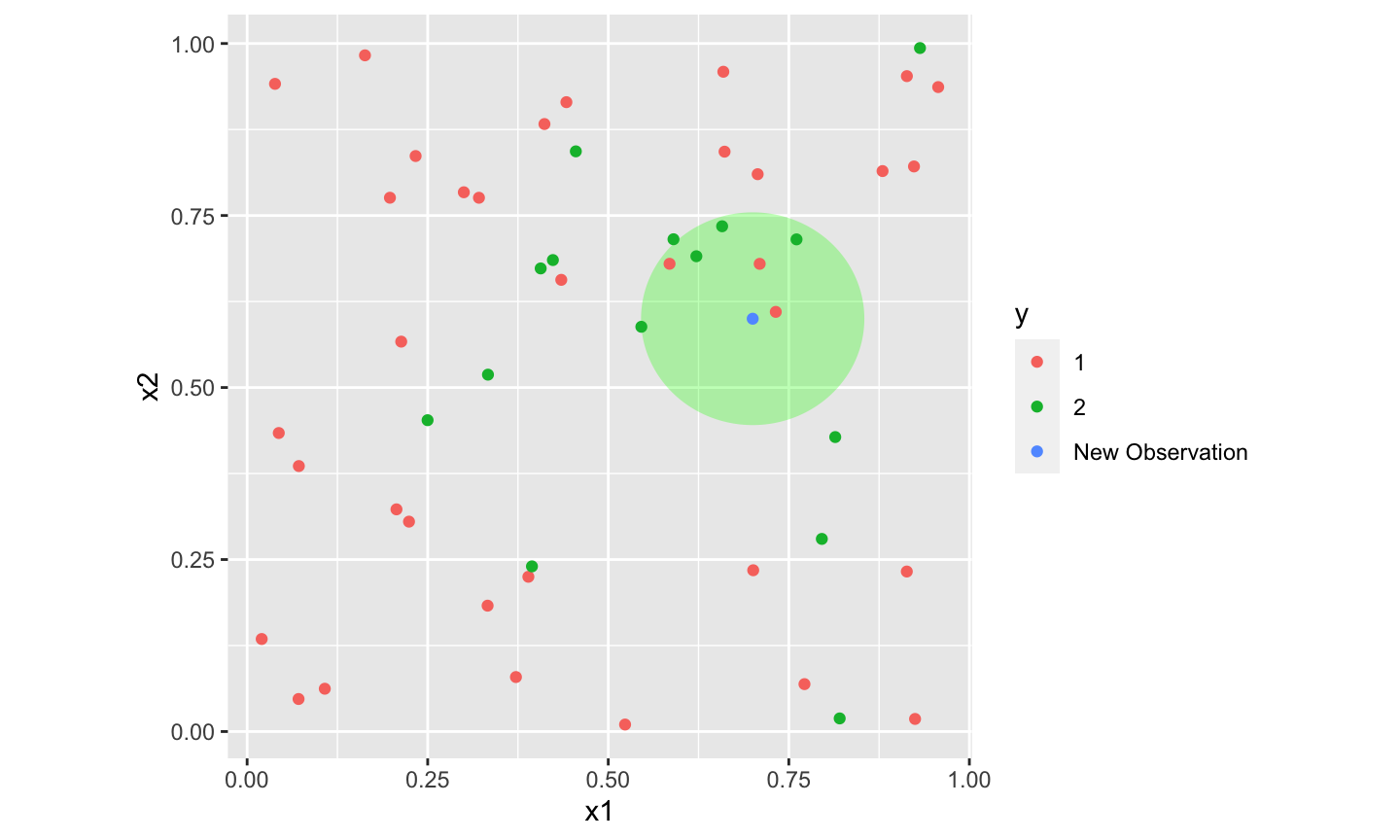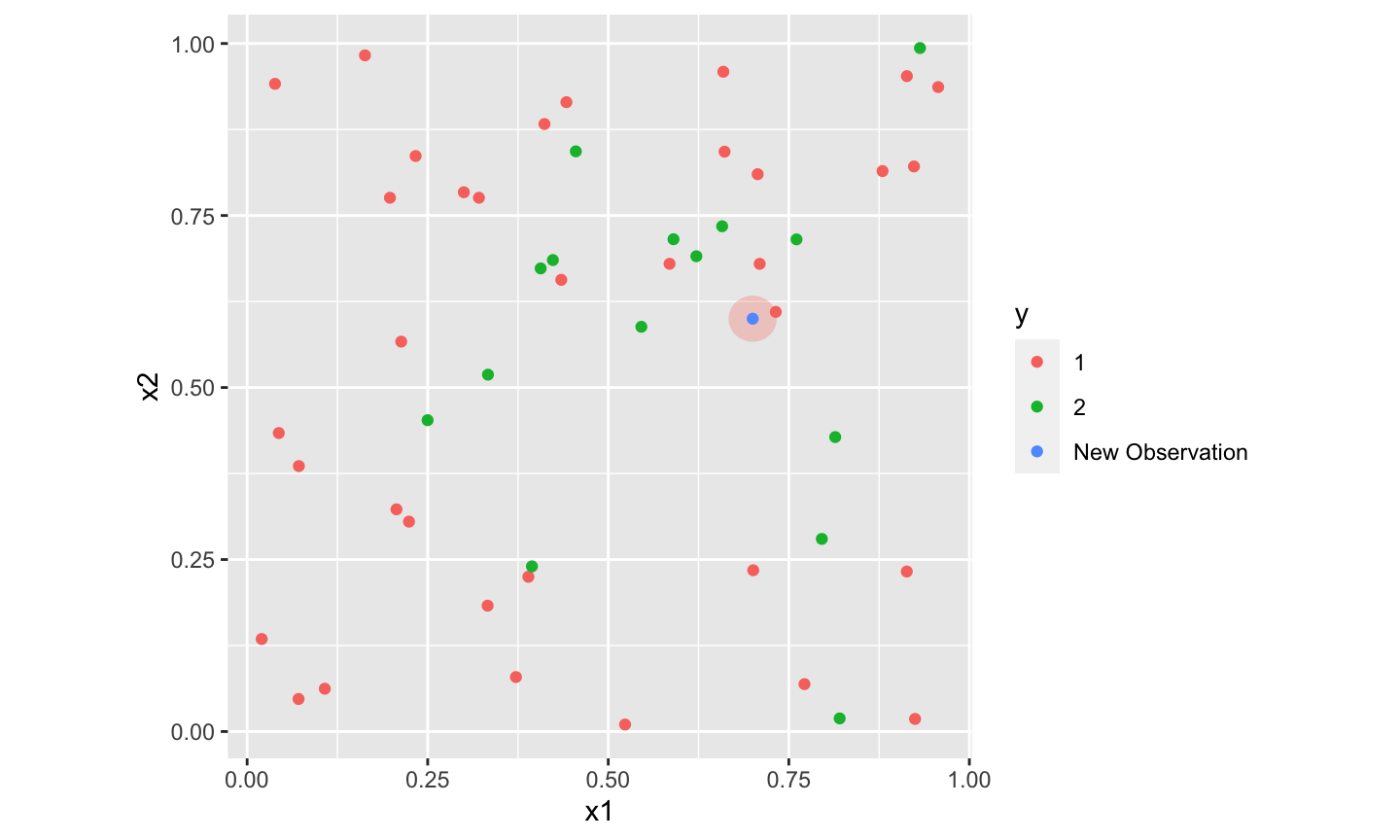April 20th, 2021

## Today’s Class

1. Review
• LASSO
• Deviance vs MSE
• Cross-Validation
• R help docs – An explainer
2. KNN
3. Binomial Classificiation: Probabilities to Predictions
4. Misclassification: Sensitivity, Specificity, ROC Curve
5. Multinomial Regression

## LASSO

The lasso penalty is the $$l_1$$ norm of our parameters: $$pen(\beta) = \sum |\beta_j|$$, which penalizes larger coefficients and non-zero coefficients. So our estimates are: $\hat{\beta}_\lambda = \underset{\beta}{\text{argmin}}\left( Dev(\beta)+\lambda \sum_{j=1}^p |\beta_j|\right)$

For a given model of the error terms, $$Dev(\beta)$$ is held fixed, so we can make comparisons within the logistic or linear regressions, but not between them easily.

When we switch logistic $$\rightarrow$$ linear, we change $$Dev$$. We can still make comparisons betwen these models but we need to ensure we are comparing the same thing.

## LASSO – MSE vs Deviance

For the logistic LASSO, our deviance is approximately: $Dev_{logit}(\beta) \propto \sum_{i=1}^n [ log(1+exp(x_i'\beta))-y_ix_i'\beta]$

While for our linear LASSO, our deviance is approximately: $Dev_{linear}(\beta) \approx {1 \over \sigma^2} \sum_{i=1}^n (y-x'\beta)^2 \propto \widehat{MSE}$

This difference derives from the different likelihoods each model uses to model their different ideas about the error distribution.

But there is only one true error distribution.

## Model Choice

When deciding which model to use, we used the out-of-sample deviance to select a value of $$\lambda$$ for each LASSO type. But we still need to choose between these two very different models.

That choice is still driven by out of sample prediction errors. We need to compare the prediction errors using the same yardstick.

So we could compare all the prediction errors using the binomial deviance, or using the MSE, or using some other loss function.

## Model Choice

Critically (and we will see this again), cross-validation gives us a good understanding of our out-of-sample error.

We can answer questions like “When I build a model in this way, what do its out of sample errors look like?”

Which means we can also answer questions like “Which model building process has better out-of-sample predictions?”

All of this is because of the very trustworthy error distributions we get from Cross-validation.

## R help documents.

I’ve realized I should give you a quick overview of how the R help documents are structured.

There are three main questions I turn to the help documents with.

1. Where in the output is $$x$$?
2. What do I put in the input to do $$y$$?
3. How do I do $$z$$?

By far, the help documents are most useful for (1) and (2).

?cv.glmnet

## Basic Structure of Help Docs

1. Description: Basic explanation of the function in question.
2. Usage: This shows the function (and close relatives), as well as most of the arguments to the function, and their defaults.
3. Arguments: This lists out every argument you can enter, describing what it must be, and what it controls.
4. Details: In-depth description of the function, often including further detail about inputs, sometimes math.
5. Value: In depth description of the output of the function.
6. Authors/Refs: People and places with even more details
7. See Also: other closely related functions. Many model tools will mention “plot, predict, and coef” methods for cv.glmnet. That implies functions like coef.cv.glmnet and plot.cv.glmnet you can look up.
8. Examples: example code running the function on basic data.

## Basic Setting

Just as in our basic prediction problems, we have data with $$n$$ observations $$(\textbf{x}_i,y_i)$$ of something.

But now $$y_i$$ is qualitative rather than quantitative. Membership in some category $$\{1,...,M\}$$

The basic problem then is the following: Given new observation covariates $$\textbf{x}_i^{new}$$, what is the class label $$y_i^{new}$$?

The quality of any classifier can be determined by its misclassification risk: $P[\hat{y}_i^{new} \neq y_i^{new}]$

## Motivation

How does this differ from basic logistic question of predicting $$P[y_i^{new}=1]$$?

1. We may have many categories, not just two.
2. We may have different discrete actions we take depending on classification.
• In some domains, as $$P[y_i=1]$$ changes, our actions change smoothly.
• e.g. as $$P[$$stock x goes up$$]$$ we buy more of it.
• In other domains, as $$P[y_i=1]$$ changes, our actions change suddenly.
• e.g. as $$P[$$get into college i$$|$$I apply$$]$$ goes up, we switch from “don’t apply” to “apply”, there is mostly no “apply a little bit more”

## Motivation

This leads to situations where we want to categorize, as it affects our decisions.

We face decisions not on a spectrum, so the most useful interpretation of our predictions may not be on that spectrum.

$$\implies$$ classification.

## Optimal Classifier

Presuming you have no preference between different types of misclassification (LOSS FUNCTION CLAIM), there is an optimal classifier, known as the Bayes Classifier.

$\hat{y}_i^{new} = \underset{j \in \{1,...,M\}}{\text{argmax}} P[y_i^{new} = j |\textbf{x}_i^{new}]$

Find the prediction which is most likely (not necessarily all that likely – e.g. $$P[\hat y = y] < 0.5$$ is common). This will minimize the misclassification risk.

## Bayes Classifier

Unfortunately, we don’t know $$P[y = j | \textbf{x}_i^{new}]$$. So the Bayes classifier is in some ways an unattainable standard.

But we can estimate it!

## Estimating P

There are many tools for estimating $$P[y|x]$$ given the training data.

• We can use parametric tools.
• Assume $$P[y|x]$$ is some function of unknown parameters $$\beta$$, estimate those, and make predictions.
• Sound familiar? Logistic Regression does this.
• We can use non-parametric tools.
• Estimate $$P[y|x]$$ directly without any parameters.
• K Nearest Neighbors (KNN)

## KNN Basics

Basic Idea: Estimate $$P[y|x]$$ locally using the labels of similar observations in the training data.

KNN: What is the most common class near $$x^{new}$$?

1. Take the $$K$$ nearest neighbors $$x_{i,1},...,x_{i,K}$$ of $$x^{new}$$ in the training data
• Nearness is (usually) Euclidean distance: $$\sqrt{\sum_{j=1}^p (x^{new}_j-x_{i,k,j})^2}$$
2. Estimate $$P[y=j|x] = \sum_{i=1}^K 1(y_i=j)$$
3. Select the class $$j$$ with the highest probability.

## KNN – Details

This (again) is sensitive to the scale of each covariate $$x$$. So we will rescale them all by standard deviations.

This will be sensitive to $$K$$, and we need to pick it.

• $$K=n$$ – we just take the mean across the entire training data.
• $$K=1$$ – Whatever observation happens to be closest will be our prediction.

Cross-validation here will help.

## KNN Example Data## KNN Example $$K=3$$## KNN Example $$K=7$$The relative ‘vote counts’ are a very crude estimate of probability.

## KNN Example $$K=1$$